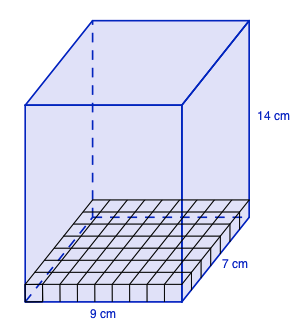### Home > GC > Chapter 9 > Lesson 9.1.3 > Problem9-30

9-30.

Draw a rectangular prism as neatly as possible on your paper. If the width is $9$ cm, the height is $14$ cm, and the depth is $7$ cm, find the surface area and volume.Use the diagram at left.

$\text{Surface Area} = 574 \; \text{cm}^2$
$\text{Volume} = 882 \; \text{cm}^3$